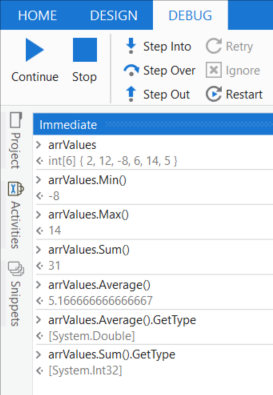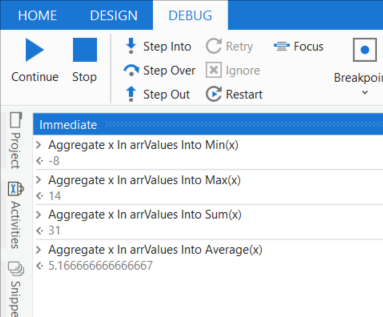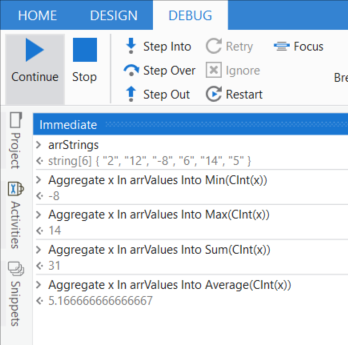# [HowTo] LINQ - First Start # Min | Max | Sum | Average Operator

This HowTo gives an introductory overview of the aggregation Operators: Min, Max, Sum, Average

# Introduction

The aggregation operators are used to retrieve from a collection a particular result by setting the contained items into a relationship.

## Overview

I – Input P – Processing O – Output
{2,12,-8,6,14,5} get the lowest value -8
{2,12,-8,6,14,5} get the highest value 14
{2,12,-8,6,14,5} sum up all values 31
{2,12,-8,6,14,5} get the average of all values 5.17

## Constraints

Performing a Min, Max, Sum, or Average operator requires that the computed values are numerical. This numerical representation of the items is

• direct given by the item’s datatype e.g.: integers: 1, decimals 2.5

OR

• by an alternate representation: e.g DateTime: Ticks, Char: Ascii Code

# Implementation

## Method Syntax

### Numericals - Short form

As mentioned above, when the processed items of a numerical datatype following short form can be used:

``````arrValues | int32( ) - an Int32 Array = {2,12,-8,6,14,5}
intResult = arrValues.Min()
intResult = arrValues.Max()
intResult = arrValues.Sum()
dblResult = arrValues.Average()
``````
Visuals### Non-Numericals - Lambda form

When the provided values are not of a numerical datatype, then a conversion function is passed to the LINQ Operator as usually done in LINQ.

```````arrStringValues | String ( ) - a String Array = {"2","12","-8","6","14","5"}`
intResult = arrValues.Sum(Function (x) CInt(x))
``````

## Query Syntax

### Appended Method approach

A common technique is to combine the Query Syntax with an appended Method Syntax, where the forwarded result value from the Query syntax block is numerical

``````arrStrings | String ( ) - a String Array = {"2","12","-8","6","14","5"}
(From x in arrStrings
Select n=Cint(x)).Min()
``````

### Aggregate / Into keywords approach

In VB.Net an equivalent can be formulated with the Aggregate and Into Assignments:

``````arrValues | int32( ) - an Int32 Array = {2,12,-8,6,14,5}
intResult = Aggregate x In arrValues Into Min(x)
intResult = Aggregate x In arrValues Into Max(x)
intResult = Aggregate x In arrValues Into Sum(x)
dblResult =  Aggregate x In arrValues Into Average(x)
``````
VisualsSimilar also the conversion can be defined:

``````arrStrings | String ( ) - a String Array = {"2","12","-8","6","14","5"}
intResult = Aggregate x In arrValues Into Min(CInt(x))
intResult = Aggregate x In arrValues Into Max(CInt(x))
intResult = Aggregate x In arrValues Into Sum(CInt(x))
dblResult = Aggregate x In arrValues Into Average(CInt(x))
``````
Visuals# Samples

## Duration string sum

``````arrDurations = { "01:15:00", "00:25:00", "00:45:00" }
totalDurationTS = Timespan.FromTicks(arrDurations.Sum(Function (x) CDate(x).TimeOfDay.Ticks))
``````

representing the timespans as a numerical value the ticks are used
from the summed-up ticks the final timespan was built

Visuals## Data table column value sum

`mySum = dtData.AsEnumerable.Sum(Function (x) CDbl(x(ColNameOrIndex).toString.Trim)`

Recommendation:
Include into the business requirements a check if non parseable values are to expect or not. Define a handling of non parseable values when it occurs like

• prechecking
• sorting out
• default value

For such a more detailed handling a LINQ statement in the Query Syntax offers a more easy to read option like

``````mySum =
(From d in dtData.AsEnumerable
Let v = d(ColNameOrIndex)
Let chk1 = Not ( isNothing(v) OrElse String.IsNullOrEmpty(v.toString.Trim))
Let chk2 = If(chk1, Double.TryParse(v.ToString, nothing), false)
Let x = If(chk2, Double.Parse(v.ToString()), 0)
Select n = x).Sum()
``````

chk1: Column value is not null or empty string
chk2: Column value passed chk1 check and is parseable (valid number string)
x: if column value cannot be parsed a default value - 0 - is set